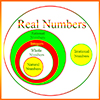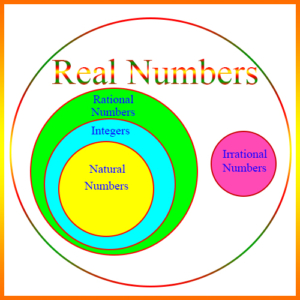# Basics of Real Numbers## Contents

Sample Real Numbers Problems

## Real Numbers – General Concepts

The set of real numbers contains all the numbers we normally deal with in real world applications. It consists of the rational numbers and the irrational numbers, as shown in the diagram.Rational numbers are all the fractions – that is, all the numbers that can be expressed as a ratio of two integers. This actually includes the integers, because every integer can be expressed as a fraction by placing 1 in the denominator. Therefore, the integers, which include the negative integers, are a subset of the rational numbers. Natural numbers are those we think of as “counting” numbers, 1, 2, 3, …, and they are a subset of the integers. Sometimes 0 is considered a natural number.

Irrational numbers are all the other real numbers. They are only called irrational because none of them can be expressed as a ratio of two integers. Examples of irrational numbers are most of the roots – for example, most of the square roots, cubed roots, 4th roots, etc. The square root of 2 is an irrational number. Indeed, the square root of any integer or rational number that is not a perfect square of some other integer or rational number is irrational. \begin{align}\pi\end{align} is also irrational. Although many think it’s equal to 3.14 (“pi day”), or to 22/7, both of these are rational numbers, and are only approximations to pi. \begin{align}\pi\end{align} is actually an infinite non-repeating decimal, and is irrational.

We normally express real numbers as integers, ratios of integers, or decimals. They can be positive or negative. They can also be very small. For example, .0000000000000000000000001 is a real number which is positive but not much bigger than 0.

Sample Real Numbers Problems

## Absolute Value of a Real Number

The absolute value of any number is the distance that number is from 0. Since distance is a positive value, the absolute value is always positive or 0, and it’s only 0 if the number is 0. Notice also that on the number line, -3 is the same distance from 0 as 3 is. Thus, the absolute value of -3, written |-3| is the same as |3|. That is, |-3| = |3|.

More generally, if x is a positive real number, then its absolute value, |x|, is x. As we said above, if x is 3, then |x| = |3| = 3. If x is a negative real number, then |x| = -x. This might look confusing, because when we see a minus sign, we think it makes the number following it negative. But this is a misconception, because if x itself is negative, then -x is positive. For example, if x is -3, then -x = 3. So, if x = -3, then |x| = |-3| = -(-3) = 3.

## Square Roots of Numbers

A number when multiplied by itself yields the number for which it is the square root. Example: 3 is the square root of 9, because 3 × 3 = 9. The square roots of most numbers are not rational numbers. For example, the square roots of 2, 3, 5, 7, etc. are real numbers, but they are not rational numbers, and therefore they are irrational numbers. Only positive numbers have square roots that are real numbers. The square roots of negative numbers are called imaginary.

### Other Roots of Numbers

Real numbers can have 3rd roots (called “cubed” roots), 4th roots, 5th roots, etc. Just as with square roots the 3rd root of a number x is a number which must be a factor of x three times. For example, the 3rd root of 8 is 2, because 2 × 2 × 2 = 8. Notice, however, that negative numbers can have 3rd roots that are real numbers. Thus, the 3rd root of -8 is -2, because -2 × -2 × -2 = -8.

Suppose we’re dealing with the nth root of a real number x, for some positive integer n. Then, if n is even, x cannot be negative, but if n is odd, then x can be negative, positive, or 0.

Sample Real Numbers Problems

## Decimal Numbers

A decimal number is a number defined using the decimal or base ten system. Its decimal point is a period that separates the integer part from the fractional part, with each numeral to the right of the decimal point representing some negative power of 10, and each numeral to the left representing some non-negative power of 10. Example:

8,765.4321

In this example, 8 is in the 1000’s (or 103) position, 7 in the 100’s (102) position, etc. 5 is in the “units” position.

To the right of the decimal point, 4 is in the tenths or 10-1 position, 3 is in the hundredths or 10-² position, etc.

If we expand this number completely, we can see the place value of all eight numerals. Thus,

8,765.4321 = 8×10³ + 7×10² + 6×10¹ + 5×100 + 4×10-1 + 3×10-2 + 2×10-3 + 1×10-4

## Decimal Arithmetic

It’s about putting the decimal point in the right place!

If decimal numbers didn’t have decimal points – in other words, if they were all integers – then addition, subtraction, multiplication, and division would simply be the same as they are for integers. Of course, we would still have the problem of dealing satisfactorily with remainders in division, but otherwise the arithmetic would be the same.

However, introducing the decimal point adds a new wrinkle. Where does the decimal point go when two decimal numbers are added, subtracted, etc.? Let’s look at each of these.

These two are basically the same – you line up the decimal points in the numbers, and you add zeros after the decimal points as needed to make the numbers the same length after the decimal point. Then you add and subtract as normal, placing the decimal point in the result immediately below its location in the numbers.

For example, suppose you want to add or subtract 24.15 and 3.222. Then lining up the decimal points and adding zeros we get:

24.150
+ 3.222
———–
27.372

24.150
– 3.222
———–
20.928

### Decimal Multiplication

In decimal multiplication you just count the number of non-zero digits to the right of the decimals in both numbers, multiply the numbers as if they were integers, and then place the decimal point in the result that many positions to the left of the end of the number.

Example: 1.1 × 1.2

There are a total of 2 digits to the right of the decimals in these two numbers. Multiplying the numbers as if they were integers, we get:

11 × 12 = 132

Now we place the decimal point in the result 2 places to the left of the end, and we get 1.32.

Thus, 1.1 × 1.2 = 1.32

### Decimal Division

Suppose you want to divide .01 by .004. Then you move the decimal points in both numbers to the right, exactly the same number of places, until both numbers are integers. Thus, we move both decimal points 3 places to the right, and we obtain 10 and 4. Now we divide 10 by 4 in the normal way we would convert a rational number into a decimal:

10/4 = 2.5

In this case, the number to the right of the decimal point terminated, because the division was exact. However, suppose we were to divide 10 by 3:

10/3 = 3.333333…

The dots at the end of the result indicate that the repeating 3’s go on indefinitely. This is a repeating decimal, and it’s an example of the decimal representation of a rational number where the decimal doesn’t terminate. All repeating decimals are in fact rational numbers. For example, the infinitely repeating decimal .428571428571428571… is actually the rational number 3/7. Usually, we show that the decimal repeats by listing all the digits until the repetition starts, and then showing dots at the end or a bar over the top of the repetitive part.

If a decimal number does not repeat, then it’s an irrational number. In the case of an irrational number, we can’t actually show what the entire number is, because its representation is infinite.

Sample Real Numbers Problems